# Schur multiplicator

Schur multiplier, of a groupThe cohomology group, where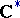is the multiplicative group of complex numbers with trivial-action. The Schur multiplicator was introduced by I. Schur  in his work on finite-dimensional complex projective representations of a group (cf. Projective representation). If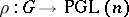is such a representation, thencan be interpreted as a mapping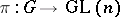such thatwhere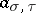is a-cocycle with values in. In particular, the projective representationis the projectivization of a linear representationif and only if the cocycledetermines the trivial element of the group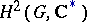. If, thenis called a closed group in the sense of Schur. Ifis a finite group, then there exist natural isomorphisms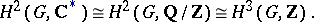Let. If a central extension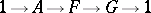(*)

of a finite groupis given, then there is a natural mapping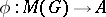whose image coincides with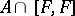. This mappingcoincides with the mapping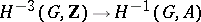induced by the cup-product with the element of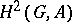defined by the extension (*). Conversely, for any subgroup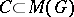there is an extension (*) such that. If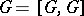, then the extension (*) is uniquely determined by the homomorphism. Ifis a monomorphism, then any projective representation ofis induced by some linear representation of.